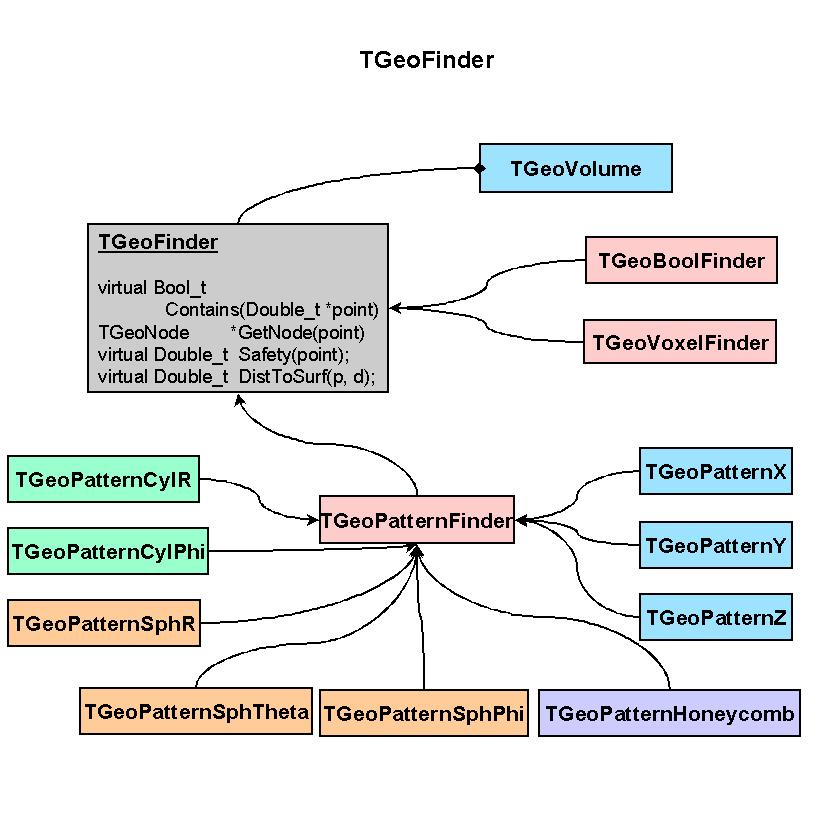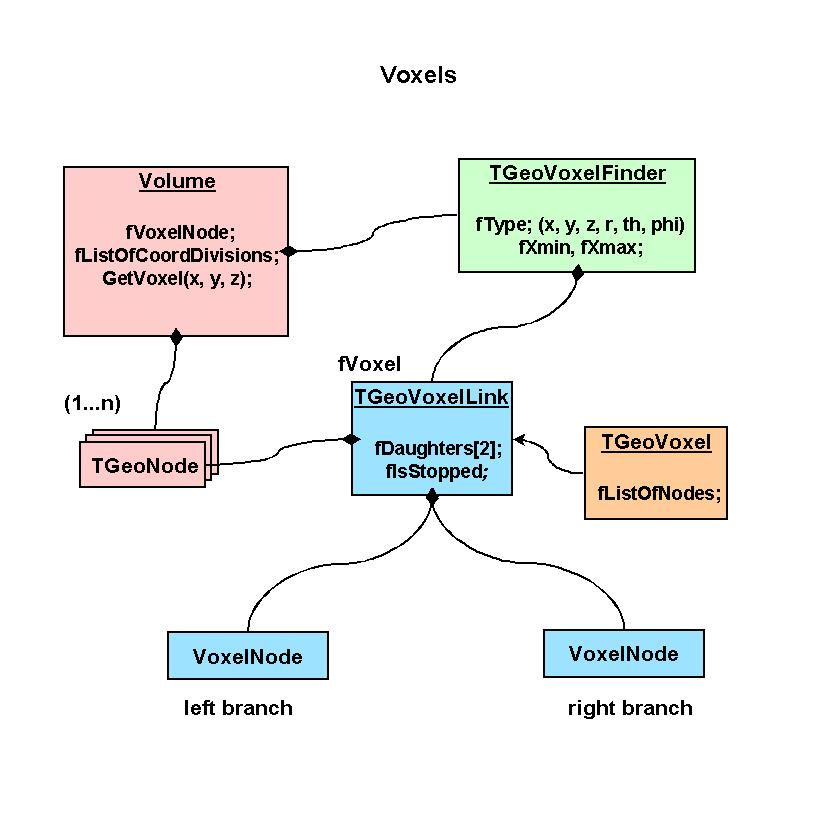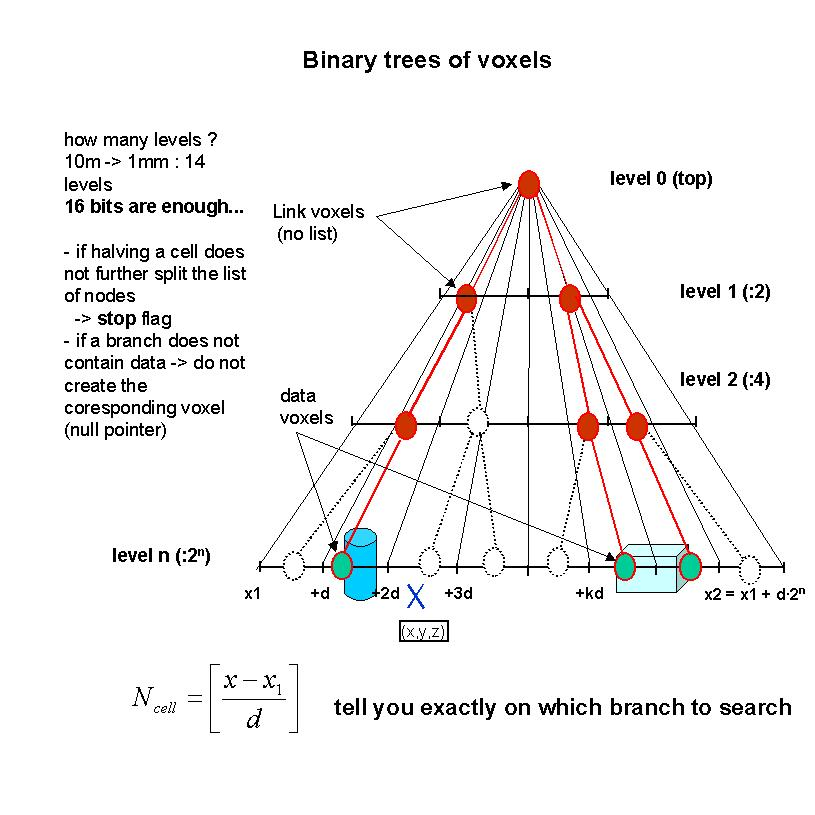library: libGeom#include "TGeoVoxelFinder.h"

# TGeoVoxelFinder

class description - source file - inheritance tree (.pdf)

## class TGeoVoxelFinder : public TObject

Inheritance Chart:
 TObject
<-
TGeoVoxelFinder
<-
 TGeoCylVoxels
 TGeoFullVoxels
```
public:
TGeoVoxelFinder()
TGeoVoxelFinder(TGeoVolume* vol)
TGeoVoxelFinder(const TGeoVoxelFinder&)
virtual ~TGeoVoxelFinder()
virtual void BuildVoxelLimits()
static TClass* Class()
void CreateCheckList()
void DaughterToMother(Int_t id, Double_t* local, Double_t* master) const
virtual Double_t Efficiency()
virtual void FindOverlaps(Int_t inode) const
Double_t* GetBoxes() const
virtual Int_t* GetCheckList(Double_t* point, Int_t& nelem)
Int_t* GetCheckList(Int_t& nelem) const
Int_t* GetExtraX(Int_t islice, Bool_t left, Int_t& nextra) const
Int_t* GetExtraY(Int_t islice, Bool_t left, Int_t& nextra) const
Int_t* GetExtraZ(Int_t islice, Bool_t left, Int_t& nextra) const
Bool_t GetIndices(Double_t* point)
Int_t GetNcandidates() const
virtual Int_t* GetNextCandidates(Double_t* point, Int_t& ncheck)
virtual Int_t* GetNextVoxel(Double_t* point, Double_t* dir, Int_t& ncheck)
Int_t GetPriority(Int_t iaxis) const
Int_t* GetValidExtra(Int_t* list, Int_t& ncheck)
Int_t* GetValidExtra(Int_t n1, UChar_t* array1, Int_t* list, Int_t& ncheck)
Int_t* GetValidExtra(Int_t n1, UChar_t* array1, Int_t n2, UChar_t* array2, Int_t* list, Int_t& ncheck)
virtual Int_t* GetVoxelCandidates(Int_t i, Int_t j, Int_t k, Int_t& ncheck)
Bool_t Intersect(Int_t n1, UChar_t* array1, Int_t& nf, Int_t* result)
Bool_t Intersect(Int_t n1, UChar_t* array1, Int_t n2, UChar_t* array2, Int_t& nf, Int_t* result)
Bool_t Intersect(Int_t n1, UChar_t* array1, Int_t n2, UChar_t* array2, Int_t n3, UChar_t* array3, Int_t& nf, Int_t* result)
Bool_t IntersectAndStore(Int_t n1, UChar_t* array1)
Bool_t IntersectAndStore(Int_t n1, UChar_t* array1, Int_t n2, UChar_t* array2)
Bool_t IntersectAndStore(Int_t n1, UChar_t* array1, Int_t n2, UChar_t* array2, Int_t n3, UChar_t* array3)
virtual TClass* IsA() const
Bool_t IsInvalid() const
Bool_t IsSafeVoxel(Double_t* point, Int_t inode, Double_t minsafe) const
TGeoVoxelFinder& operator=(const TGeoVoxelFinder&)
virtual void Print(Option_t* option) const
void PrintVoxelLimits(Double_t* point) const
void SetInvalid(Bool_t flag = kTRUE)
virtual void ShowMembers(TMemberInspector& insp, char* parent)
virtual void SortAll(Option_t* option)
void SortCrossedVoxels(Double_t* point, Double_t* dir)
virtual void Streamer(TBuffer& b)
void StreamerNVirtual(TBuffer& b)
Bool_t Union(Int_t n1, UChar_t* array1)
Bool_t Union(Int_t n1, UChar_t* array1, Int_t n2, UChar_t* array2)
Bool_t Union(Int_t n1, UChar_t* array1, Int_t n2, UChar_t* array2, Int_t n3, UChar_t* array3)
virtual void Voxelize(Option_t* option)
```

### Data Members

```
protected:
TGeoVolume* fVolume        volume to which applies
Int_t fNcandidates   ! number of candidates
Int_t fCurrentVoxel  ! index of current voxel in sorted list
Int_t fIbx           number of different boundaries on X axis
Int_t fIby           number of different boundaries on Y axis
Int_t fIbz           number of different boundaries on Z axis
Int_t fNboxes        length of boxes array
Int_t fNox           length of array of X offsets
Int_t fNoy           length of array of Y offsets
Int_t fNoz           length of array of Z offsets
Int_t fNex           length of array of X extra offsets
Int_t fNey           length of array of Y extra offsets
Int_t fNez           length of array of Z extra offsets
Int_t fNx            length of array of X voxels
Int_t fNy            length of array of Y voxels
Int_t fNz            length of array of Z voxels
Int_t fPriority   priority for each axis
Int_t fSlices     ! slice indices for current voxel
Int_t fInc        ! slice index increment
Double_t fInvdir     ! 1/current director cosines
Double_t fLimits     limits on X,Y,Z
Double_t* fBoxes         [fNboxes] list of bounding boxes
Double_t* fXb            [fIbx] ordered array of X box boundaries
Double_t* fYb            [fIby] ordered array of Y box boundaries
Double_t* fZb            [fIbz] ordered array of Z box boundaries
Int_t* fOBx           [fNox] offsets of daughter indices for slices X
Int_t* fOBy           [fNoy] offsets of daughter indices for slices Y
Int_t* fOBz           [fNoz] offsets of daughter indices for slices Z
Int_t* fOEx           [fNox] offsets of extra indices for slices X
Int_t* fOEy           [fNoy] offsets of extra indices for slices Y
Int_t* fOEz           [fNoz] offsets of extra indices for slices Z
Int_t* fIndX          [fNx] indices of daughters inside boundaries X
Int_t* fIndY          [fNy] indices of daughters inside boundaries Y
Int_t* fIndZ          [fNz] indices of daughters inside boundaries Z
Int_t* fExtraX        [fNex] indices of extra daughters in X slices
Int_t* fExtraY        [fNey] indices of extra daughters in Y slices
Int_t* fExtraZ        [fNez] indices of extra daughters in Z slices
Int_t* fCheckList     ! list of candidates

public:
static const TGeoVoxelFinder::EVoxelsType kGeoInvalidVoxels
```

## Class Description

``` Full description with examples and pictures

```
/**/

TGeoVoxelFinder()
``` Default constructor
```

TGeoVoxelFinder(TGeoVolume *vol)
``` Default constructor
```

~TGeoVoxelFinder()
``` Destructor
printf("deleting finder of %s\n", fVolume->GetName());
```

void BuildVoxelLimits()
``` build the array of bounding boxes of the nodes inside
```

void CreateCheckList()
``` Initializes check list.
```

void DaughterToMother(Int_t id, Double_t *local, Double_t *master) const
``` convert a point from the local reference system of node id to reference
system of mother volume
```

Bool_t IsSafeVoxel(Double_t *point, Int_t inode, Double_t minsafe) const
``` Computes squared distance from POINT to the voxel(s) containing node INODE. Returns 0
if POINT inside voxel(s).
```

Double_t Efficiency()
```--- Compute voxelization efficiency.
```

void FindOverlaps(Int_t inode) const
``` create the list of nodes for which the bboxes overlap with inode's bbox
```

Bool_t GetIndices(Double_t *point)
``` Getindices for current slices on x, y, z
```

Int_t* GetExtraX(Int_t islice, Bool_t left, Int_t &nextra) const
```--- Return the list of extra candidates in a given X slice compared to
another (left or right)
```

Int_t* GetExtraY(Int_t islice, Bool_t left, Int_t &nextra) const
```--- Return the list of extra candidates in a given Y slice compared to
another (left or right)
```

Int_t* GetExtraZ(Int_t islice, Bool_t left, Int_t &nextra) const
```--- Return the list of extra candidates in a given Z slice compared to
another (left or right)
```

Int_t* GetValidExtra(Int_t *list, Int_t &ncheck)
``` Get extra candidates that are not contained in current check list
```

Int_t* GetValidExtra(Int_t /*n1*/, UChar_t *array1, Int_t *list, Int_t &ncheck)
``` Get extra candidates that are contained in array1 but not in current check list
```

Int_t* GetValidExtra(Int_t /*n1*/, UChar_t *array1, Int_t /*n2*/, UChar_t *array2, Int_t *list, Int_t &ncheck)
``` Get extra candidates that are contained in array1 but not in current check list
```

Int_t* GetNextCandidates(Double_t *point, Int_t &ncheck)
``` Returns list of new candidates in next voxel. If NULL, nowhere to
go next.
```

void SortCrossedVoxels(Double_t *point, Double_t *dir)
``` get the list in the next voxel crossed by a ray
```

Int_t* GetCheckList(Double_t *point, Int_t &nelem)
``` get the list of daughter indices for which point is inside their bbox
if (!fBoxes) return 0;
```

Int_t* GetVoxelCandidates(Int_t i, Int_t j, Int_t k, Int_t &ncheck)
``` get the list of candidates in voxel (i,j,k) - no check
```

Int_t* GetNextVoxel(Double_t *point, Double_t * /*dir*/, Int_t &ncheck)
``` get the list of new candidates for the next voxel crossed by current ray
printf("### GetNextVoxel\n");
```

Bool_t Intersect(Int_t n1, UChar_t *array1, Int_t &nf, Int_t *result)
``` return the list of nodes corresponding to one array of bits
```

Bool_t IntersectAndStore(Int_t n1, UChar_t *array1)
``` return the list of nodes corresponding to one array of bits
```

Bool_t Union(Int_t n1, UChar_t *array1)
``` make union of older bits with new array
printf("Union - one slice\n");
```

Bool_t Union(Int_t /*n1*/, UChar_t *array1, Int_t /*n2*/, UChar_t *array2)
``` make union of older bits with new array
printf("Union - two slices\n");
```

Bool_t Union(Int_t /*n1*/, UChar_t *array1, Int_t /*n2*/, UChar_t *array2, Int_t /*n3*/, UChar_t *array3)
``` make union of older bits with new array
printf("Union - three slices\n");
printf("n1=%i n2=%i n3=%i\n", n1,n2,n3);
```

Bool_t Intersect(Int_t n1, UChar_t *array1, Int_t n2, UChar_t *array2, Int_t &nf, Int_t *result)
``` return the list of nodes corresponding to the intersection of two arrays of bits
```

Bool_t IntersectAndStore(Int_t /*n1*/, UChar_t *array1, Int_t /*n2*/, UChar_t *array2)
``` return the list of nodes corresponding to the intersection of two arrays of bits
```

Bool_t Intersect(Int_t n1, UChar_t *array1, Int_t n2, UChar_t *array2, Int_t n3, UChar_t *array3, Int_t &nf, Int_t *result)
``` return the list of nodes corresponding to the intersection of three arrays of bits
```

Bool_t IntersectAndStore(Int_t /*n1*/, UChar_t *array1, Int_t /*n2*/, UChar_t *array2, Int_t /*n3*/, UChar_t *array3)
``` return the list of nodes corresponding to the intersection of three arrays of bits
```

void SortAll(Option_t *)
``` order bounding boxes along x, y, z
```

void Print(Option_t *) const

void PrintVoxelLimits(Double_t *point) const
``` print the voxel containing point
```

void Voxelize(Option_t * /*option*/)
``` Voxelize attached volume according to option
```

### Inline Functions

```                  Int_t* GetCheckList(Int_t& nelem) const
Int_t GetPriority(Int_t iaxis) const
Int_t GetNcandidates() const
Bool_t IsInvalid() const
Double_t* GetBoxes() const
void SetInvalid(Bool_t flag = kTRUE)
TClass* Class()
TClass* IsA() const
void ShowMembers(TMemberInspector& insp, char* parent)
void Streamer(TBuffer& b)
void StreamerNVirtual(TBuffer& b)
TGeoVoxelFinder TGeoVoxelFinder(const TGeoVoxelFinder&)
TGeoVoxelFinder& operator=(const TGeoVoxelFinder&)
```

Author: Andrei Gheata 04/02/02
Last update: root/geom:\$Name: \$:\$Id: TGeoVoxelFinder.cxx,v 1.22 2004/01/20 15:44:33 brun Exp \$